太棒了！Seaborn还可以在一行代码中进行各种回归分析,厉害,也,能,做,多种,统统,只,需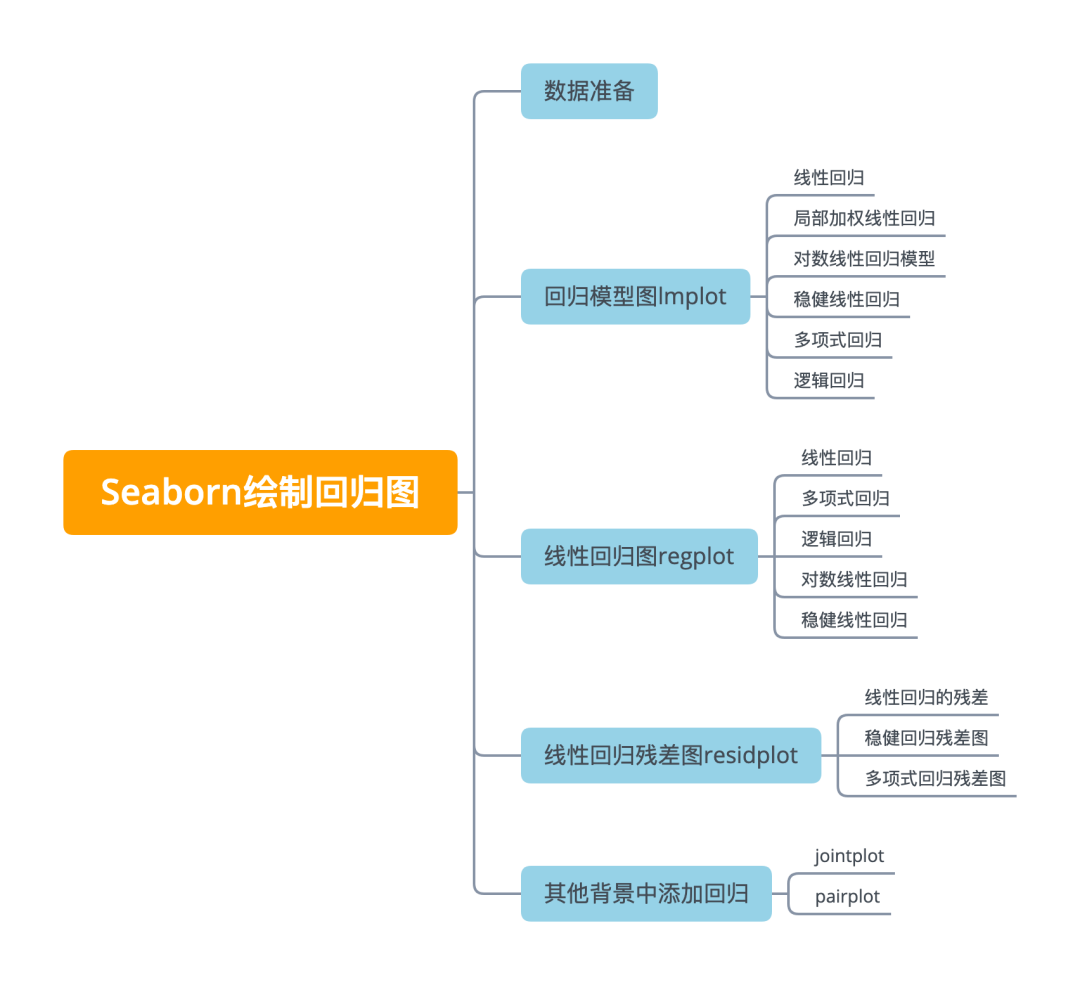数据准备

import numpy as np
import matplotlib.pyplot as plt
import pandas as pd
import seaborn as sns
import baostock as bs
result = bs.query_history_k_data('sh.601318',
fields = 'date,open,high, low,close,volume',
start_date = '2020-01-01',
end_date = '2021-05-01',
frequency='d')
dataset = result.get_data().set_index('date').applymap(lambda x: float(x))
bs.logout()
dataset['Open_Close'] = (dataset['open'] - dataset['close'])/dataset['open']
dataset['High_Low'] = (dataset['high'] - dataset['low'])/dataset['low']
dataset['Increase_Decrease'] = np.where(dataset['volume'].shift(-1) > dataset['volume'],1,0)
dataset['Returns'] = dataset['close'].pct_change()
dataset = dataset.dropna()
dataset['Up_Down'] = np.where(dataset['Returns'].shift(-1) > dataset['Returns'],'Up','Down')
dataset = dataset.dropna()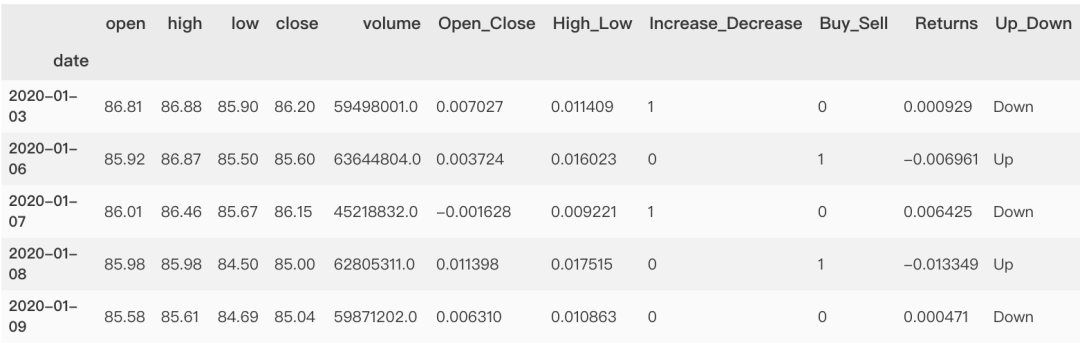回归模型图 lmplot

lmplot 是一种集合基础绘图与基于数据建立回归模型的绘图方法。通过 lmplot 我们可以直观地总览数据的内在关系。显示每个数据集的线性回归结果， xy 变量，利用 'hue'、'col'、'row' 参数来控制绘图变量。可以把它看作分类绘图依据。

线性回归

l mplot 绘制散点图及线性回归拟合线非常简单，只需要指定自变量和因变量即可， lmplot 会自动完成线性回归拟合。回归模型的置信区间用回归线周围的半透明带绘制。

lmplot 支持引入第三维度进行对比，例如我们设置 hue="species"

sns.lmplot(x="open",
y="close",
hue="Up_Down",
data=dataset)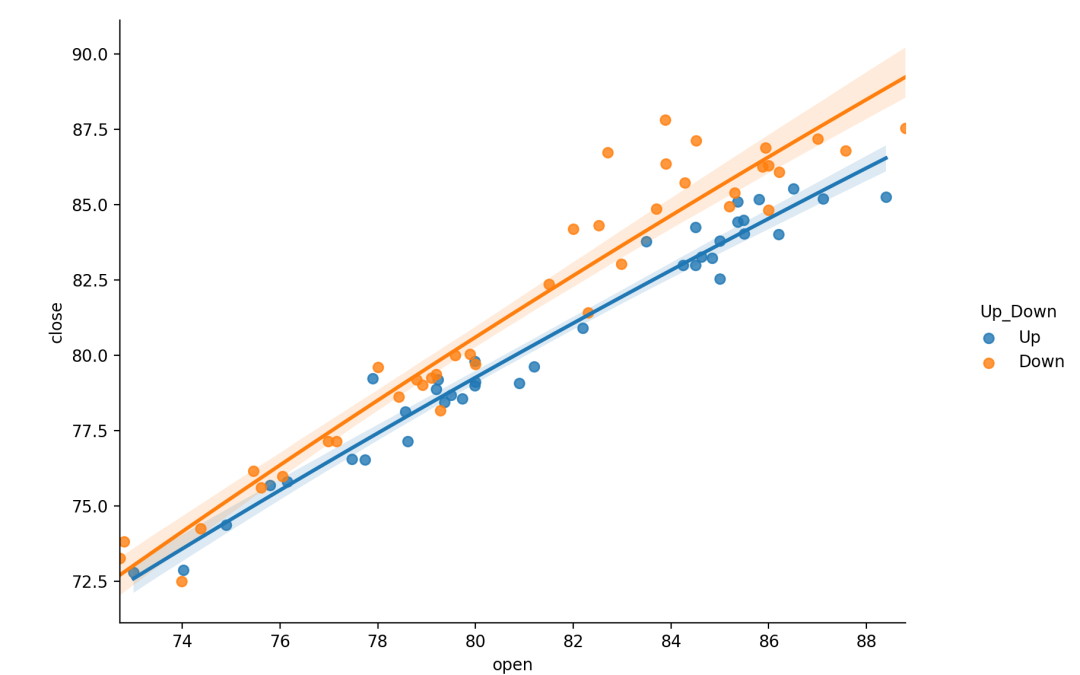局部加权线性回归

lowess bool, 可选

sns.lmplot(x="open",
y="close",
hue="Up_Down",
lowess=True,
data=dataset)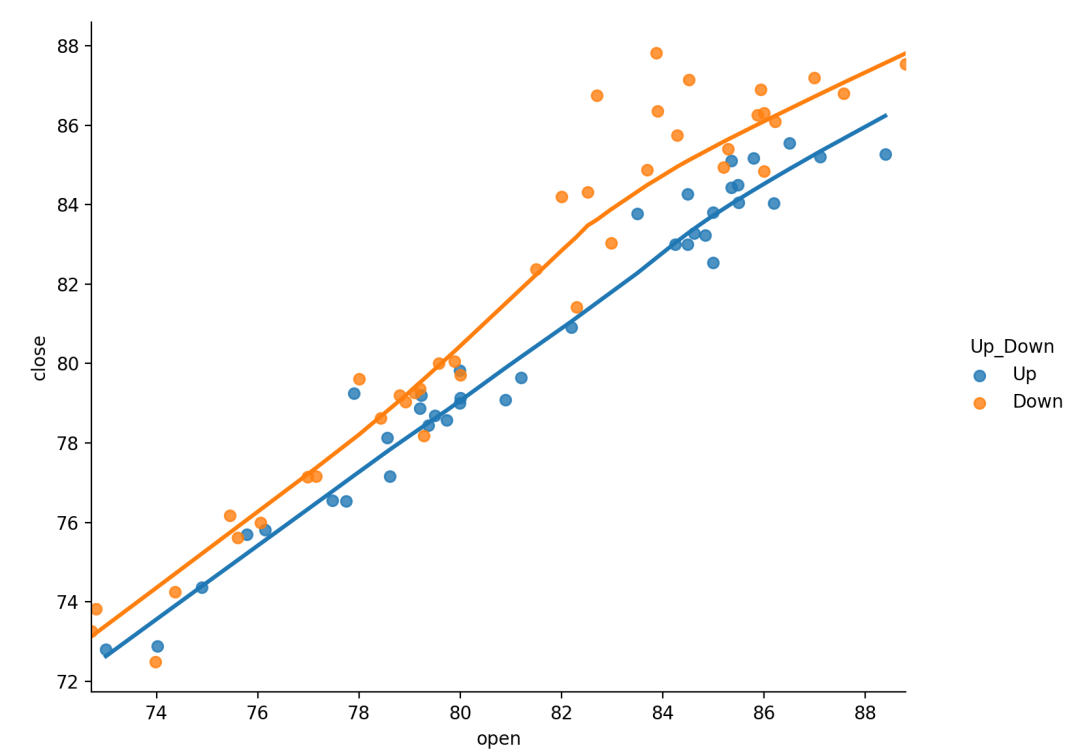对数线性回归模型

logx : bool, 可选

sns.lmplot(x="open",
y="close",
hue="Up_Down",
data=dataset,
logx=True)稳健线性回归

hue, col, row : strings

height : scalar, 可选

col_wrap : int, 可选

n_boot int, 可选

ci int in [ 0，100 ]或None, 可选

robust bool, 可选

sns.lmplot(x="open",
y="volume",
data=dataset,
hue="Increase_Decrease",
col="Increase_Decrease",
# col|hue控制子图不同的变量species
col_wrap=2,
height=4,
robust=True)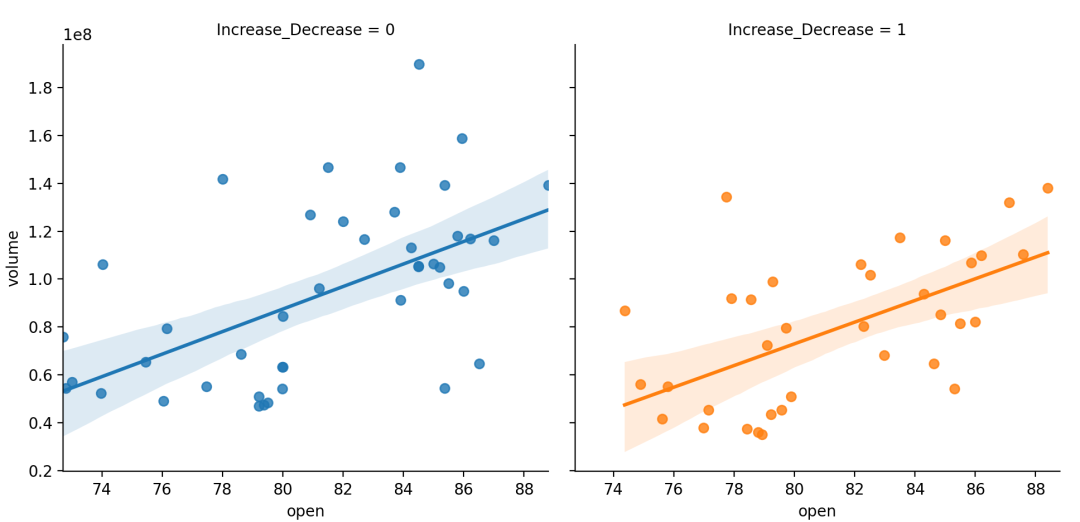多项式回归

order : int, 可选

sns.lmplot(x="close",
y="volume",
data=dataset,
hue="Increase_Decrease",
col="Up_Down", # col|hue控制子图不同的变量species
col_wrap=2,    # col_wrap控制每行子图数量
height=4,      # height控制子图高度
order=3        # 多项式最高次幂
)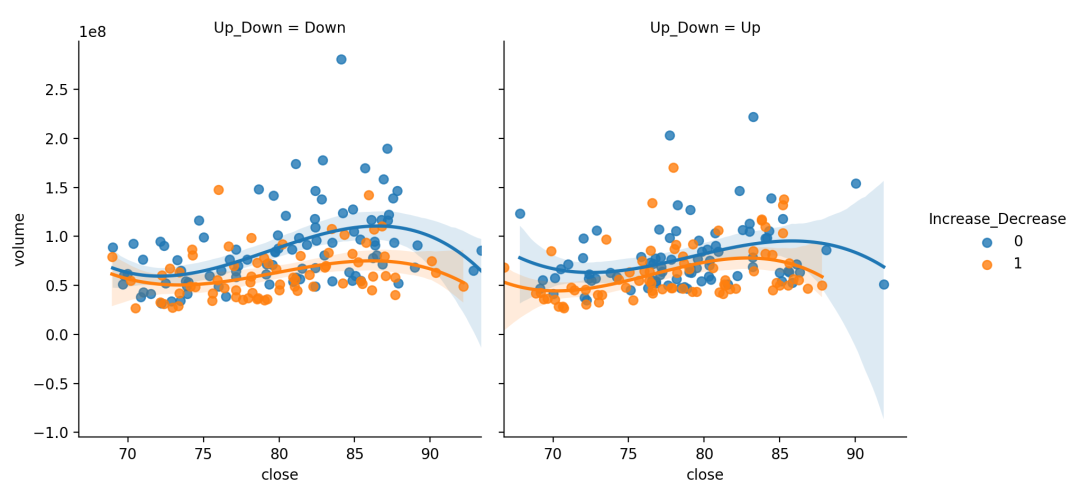逻辑回归

Logistic 回归是一种广义线性回归， logistic 回归的因变量可以是二分类的，也可以是多分类的，但是二分类的更为常用，也更加容易解释，多类可以使用 softmax 方法进行处理。

{x,y}_jitter floats, 可选

logistic bool, 可选

# 制作具有性别色彩的自定义调色板
pal = dict(Up= "#6495ED", Down= "#F08080")
# 买卖随开盘价与涨跌变化
g = sns.lmplot(x= "open", y= "Buy_Sell", col= "Up_Down", hue= "Up_Down",
data=dataset,
palette=pal,
y_jitter= .02, # 回归噪声
logistic= True)# 逻辑回归模型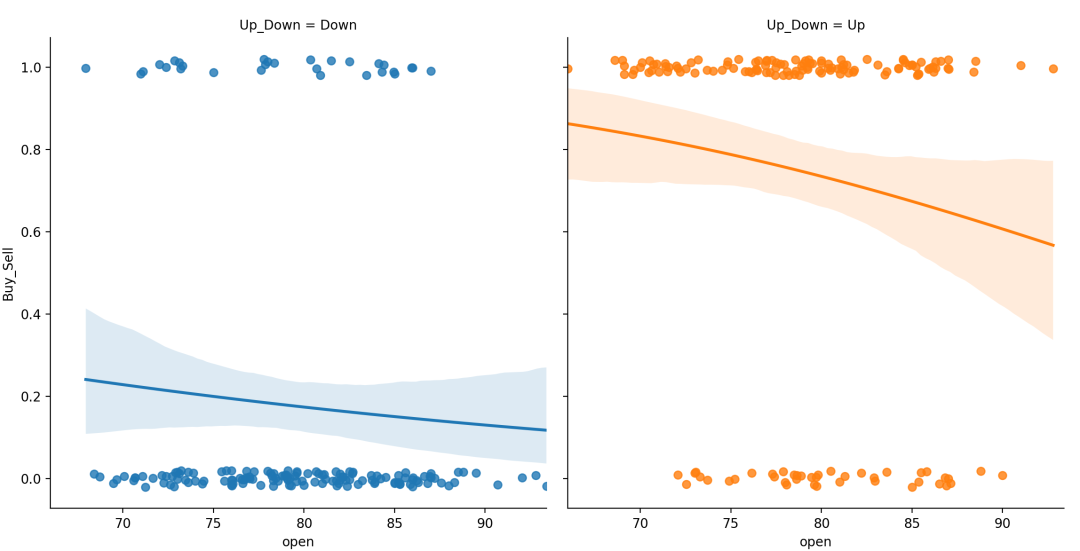线性回归图 regplot

Lmplot() regplot() 与两个函数之间的主要区别是 regplot() 接受变量 的类型可以是 numpy数组、pandas序列(Series) 。或者直接对 data传入pandas DataFrame 对象数据。而 lmplot() data 参数是必须的，且变量 必须为字符串。

线性回归

fit_reg bool，可选

ci int in [ 0，100 ]或None，可选

scatter bool，可选

# 绘制线性回归拟合曲线
f, ax = plt.subplots(figsize=(8,6))
sns.regplot(x="Returns",
y="volume",
data=dataset,
fit_reg=True,
ci = 95,
scatter=True,
ax=ax)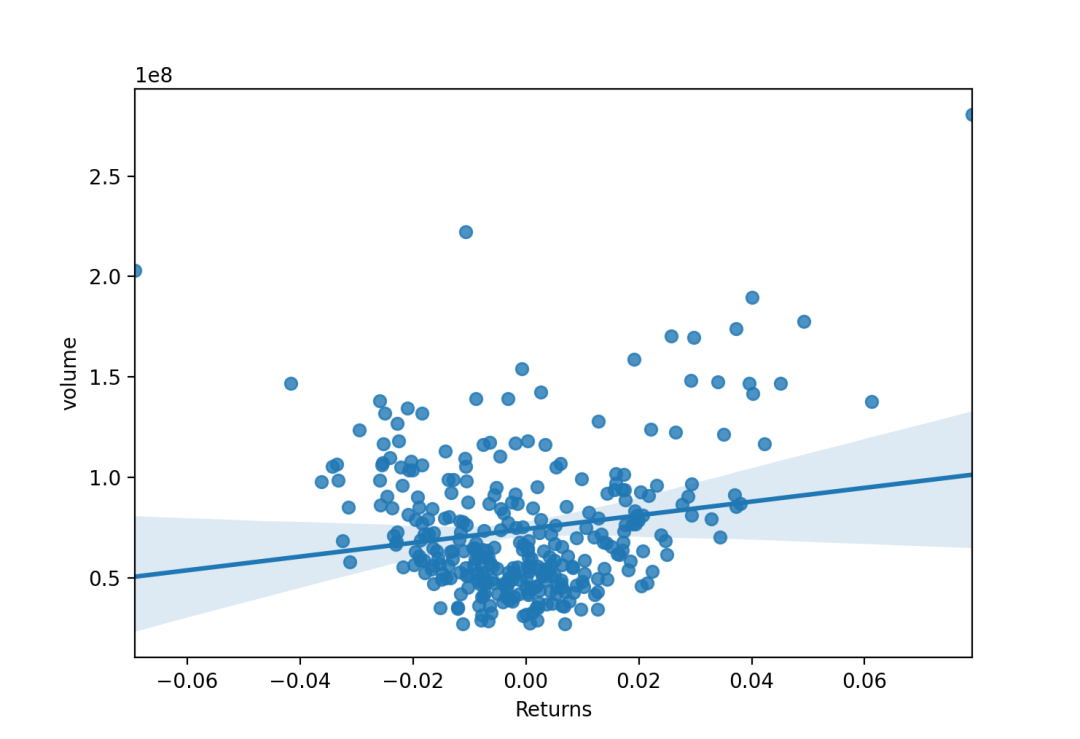x_estimator callable映射向量->标量，可选

x_bins int或vector，可选
x 变量分为离散的 bin ，然后估计中心趋势和置信区间。这种装箱仅影响散点图的绘制方式；回归仍然适合原始数据。该参数可以解释为均匀大小（不必要间隔）的垃圾箱数或垃圾箱中心的位置。使用此参数时，表示默认 x_estimator numpy.mean

x_ci “ ci”，“ sd”，[ 0，100 ]中的int或None，可选

f, ax = plt.subplots(1,2,figsize=(15,6))
sns.regplot(x="Returns",
y="volume",
data=dataset,
x_bins=10,
x_ci="ci",
ax=ax)
# 带有离散x变量的图，显示了唯一值的方差和置信区间：
sns.regplot(x="Returns",
y="volume",
data=dataset,
x_bins=10,
x_ci='sd',
ax=ax)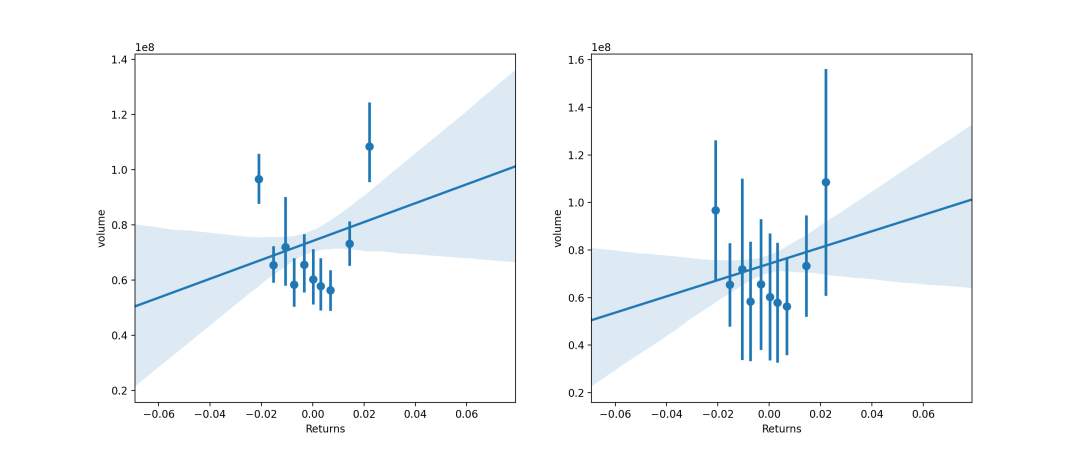多项式回归

order : int, 可选

sns.regplot(x="open",
y="close",
data=dataset.loc[dataset.Up_Down == "Up"],
scatter_kws={"s": 80},
order=5, ci=None)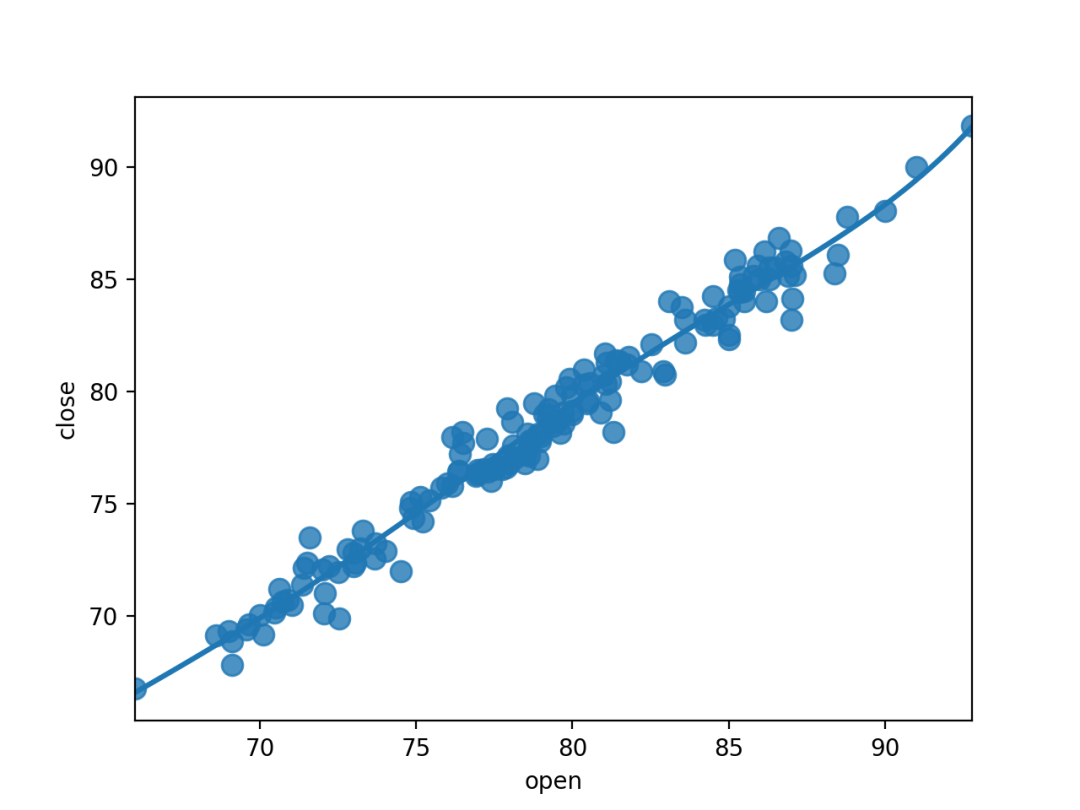逻辑回归

{x,y}_jitter floats, 可选

n_boot int, 可选

sns.regplot(x= "volume",
y= "Increase_Decrease",
data=dataset,
logistic=True,
n_boot=500,
y_jitter=.03,)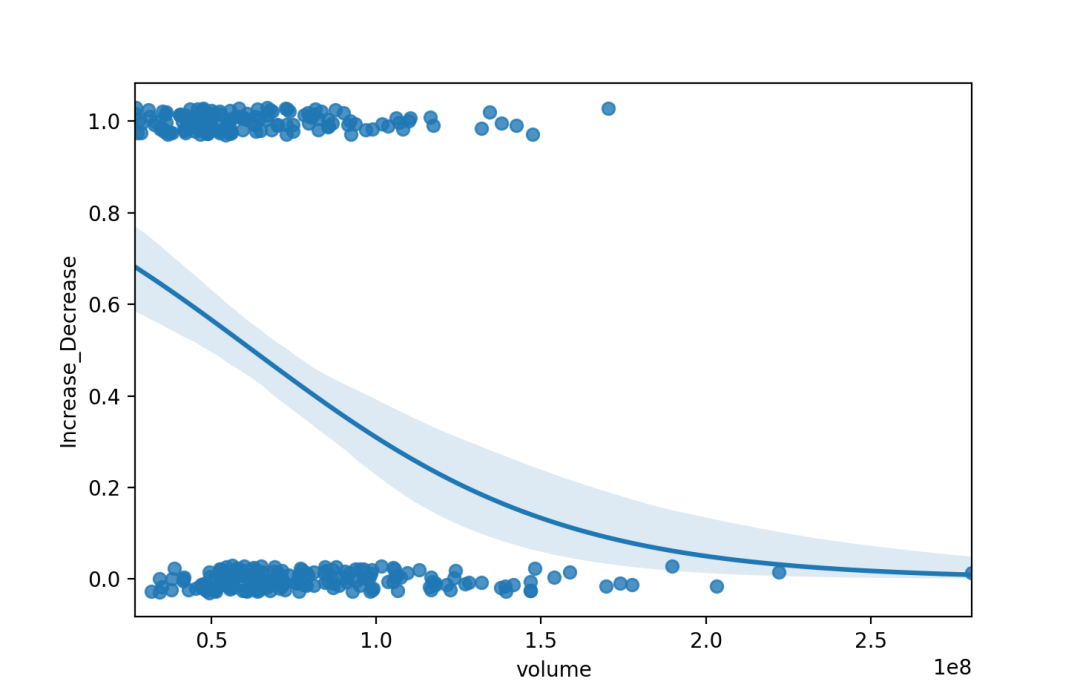对数线性回归

logx bool, 可选

sns.regplot(x="open",
y="volume",
data=dataset.loc[dataset.Up_Down == "Up"],
x_estimator=np.mean,
logx=True)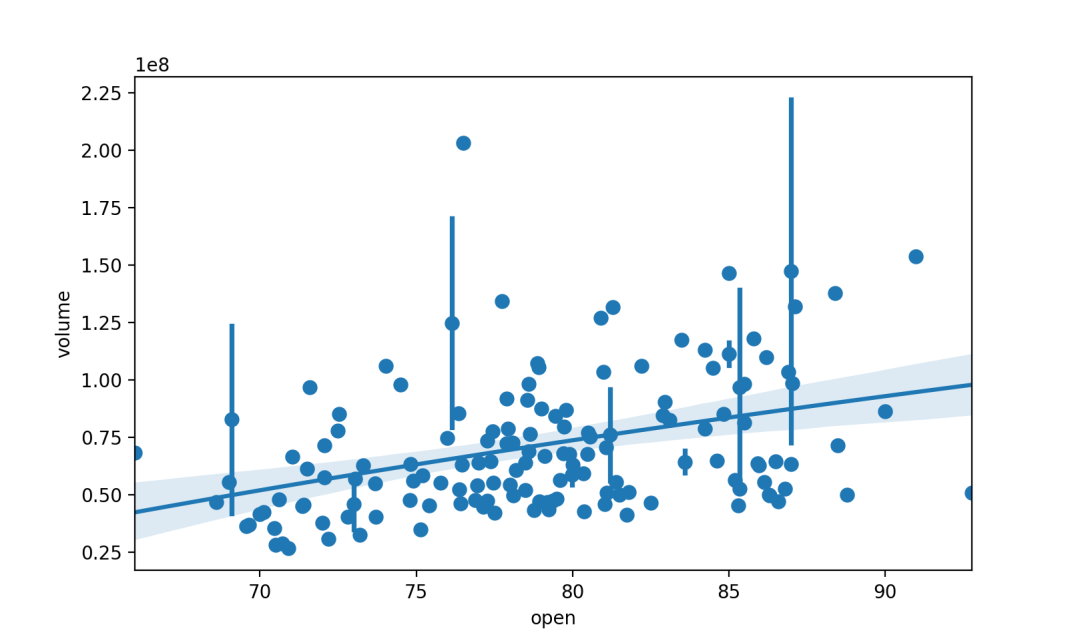稳健线性回归

robust 布尔值，可选

sns.regplot(x="open",
y="Returns",
data=dataset.loc[dataset.Up_Down == "Up"],
scatter_kws={"s": 80},
robust=True, ci=None)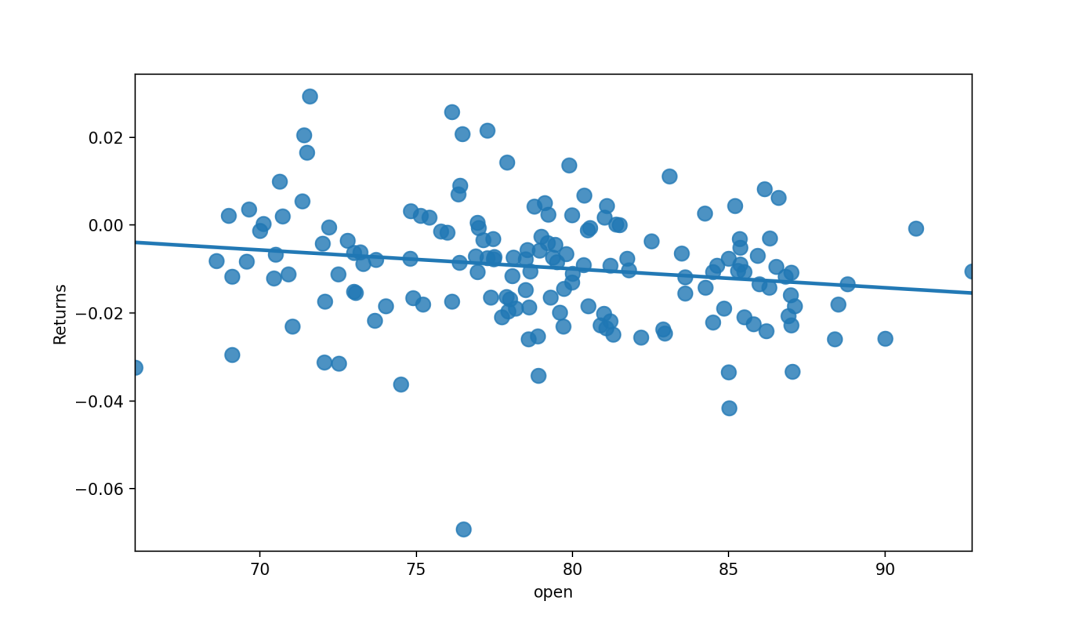线性回归残差图residplot

residplot() 用于检查简单的回归模型是否拟合数据集。它拟合并移除一个简单的线性回归，然后绘制每个观察值的残差值。通过观察数据的残差分布是否具有结构性，若有则这意味着我们当前选择的模型不是很适合。

线性回归的残差

lowess 布尔值，可选

# 拟合线性模型后绘制残差,lowess平滑
x=dataset.open
y=dataset.Returns
sns.residplot(x=x, y=y,
lowess=True,
color="g")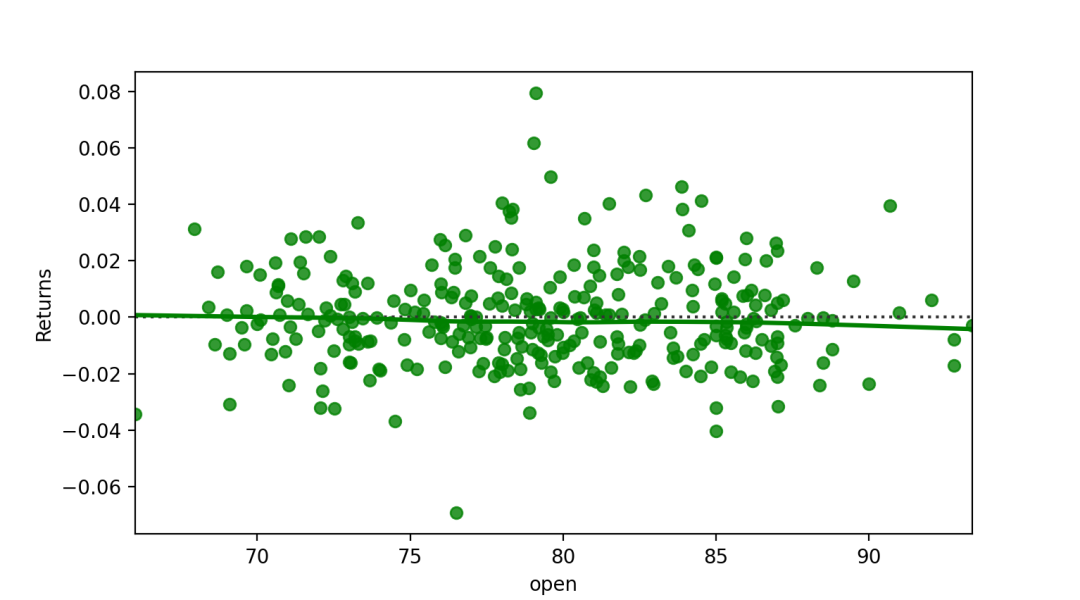稳健回归残差图

robust bool，可选

sns.residplot(x="open",
y="Returns",
data=dataset.loc[dataset.Up_Down == "Up"],
robust=True,
lowess=True)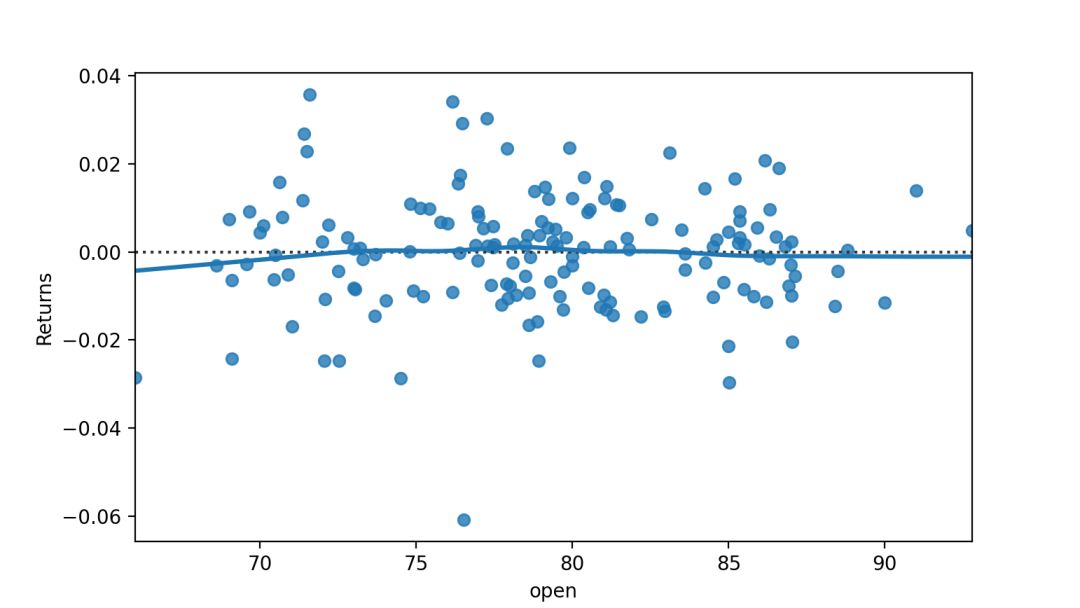多项式回归残差图

order int，可选

sns.residplot(x="open",
y="close",
data=dataset.loc[dataset.Up_Down == "Up"],
order=3,
lowess=True)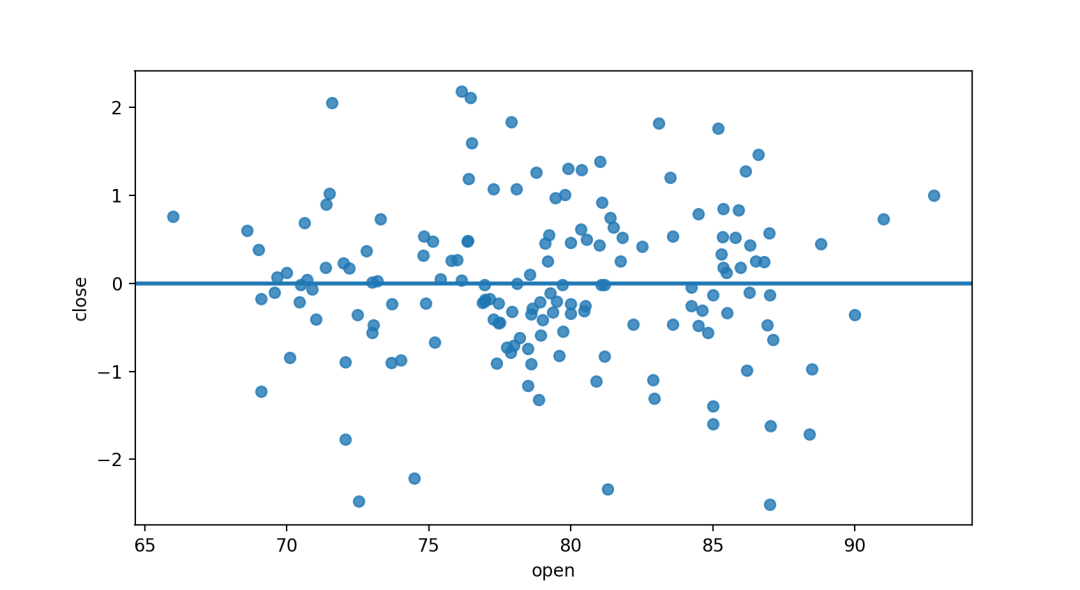其他背景中添加回归

jointplot

jointplot() 函数在其他更大、更复杂的图形背景中使用 regplot() jointplot() 可以通过 kind="reg" 来调用 regplot() 绘制线性关系。

sns.jointplot("open",
"Returns",
data=dataset,
kind='reg')
# 设置kind="reg"为添加线性回归拟合
#（使用regplot()）和单变量KDE曲线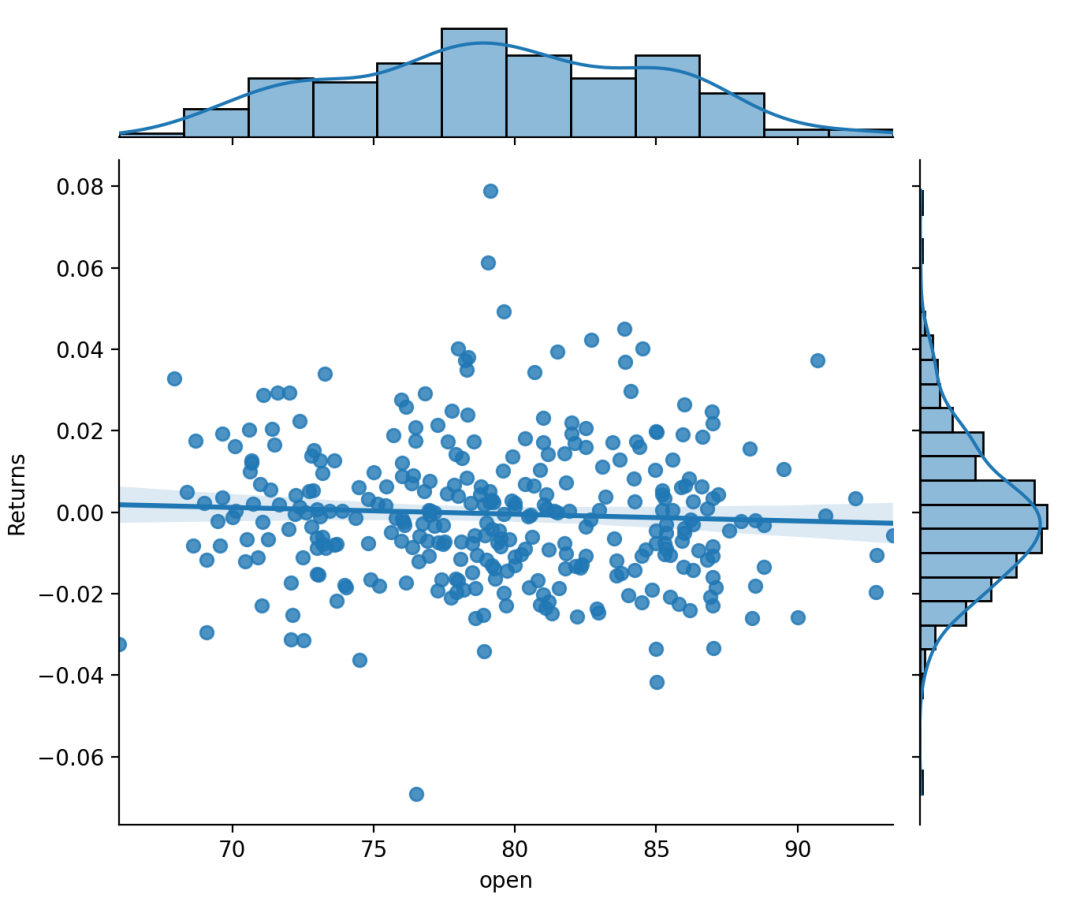jointplot() 可以通过 kind="resid" 来调用 residplot() 绘制具有单变量边际分布。

sns.jointplot(x="open",
y="close",
data=dataset,
kind="resid")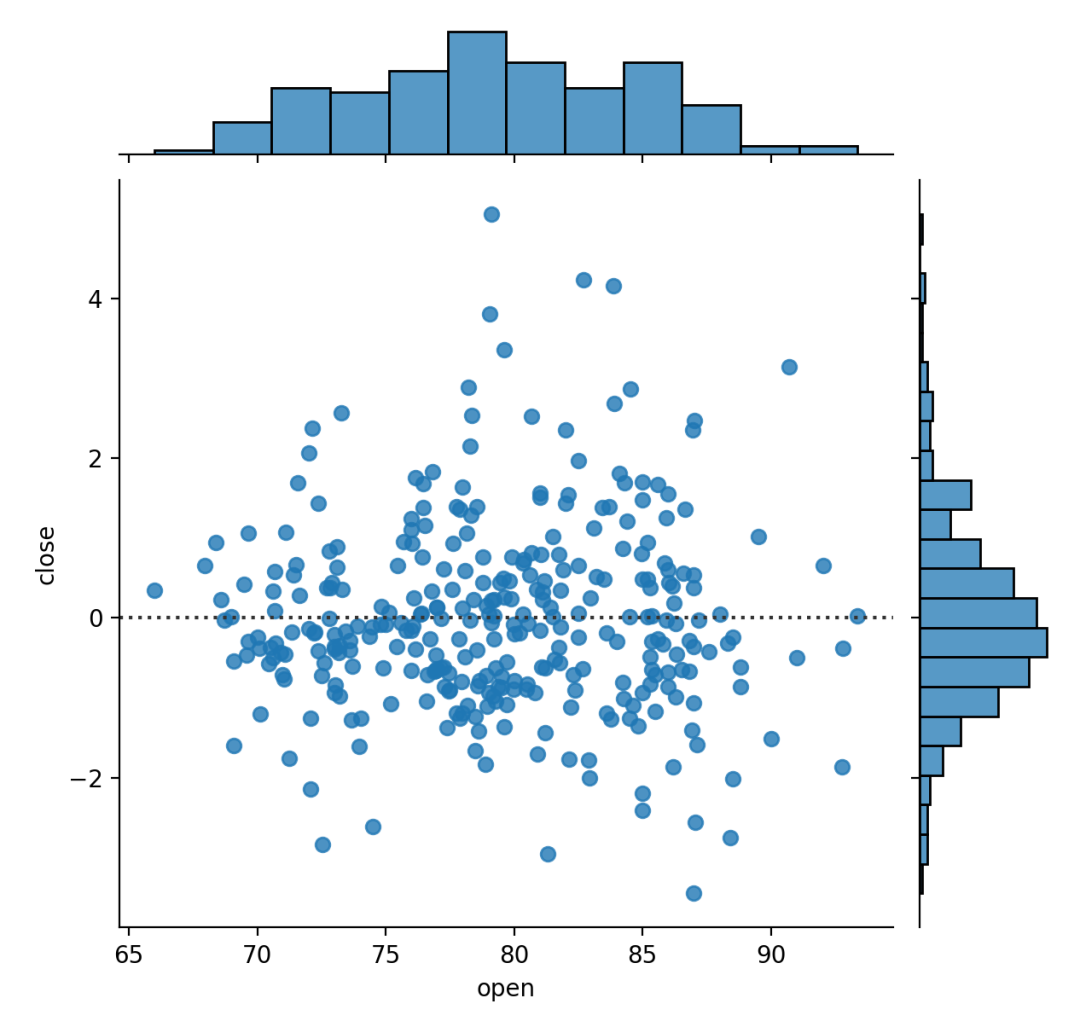pairplot

pairplot() 传入 kind="reg" 参数则会融合 regplot() PairGrid 来展示变量间的线性关系。注意这里和 lmplot() 的区别， lmplot() 绘制的行（或列）是将一个变量的多个水平（分类、取值）展开，而在这里， PairGrid 则是绘制了不同变量之间的线性关系。

sns.pairplot(dataset,
x_vars=["open", "close"],
y_vars=["Returns"],
height=5,
aspect=.8,
kind="reg");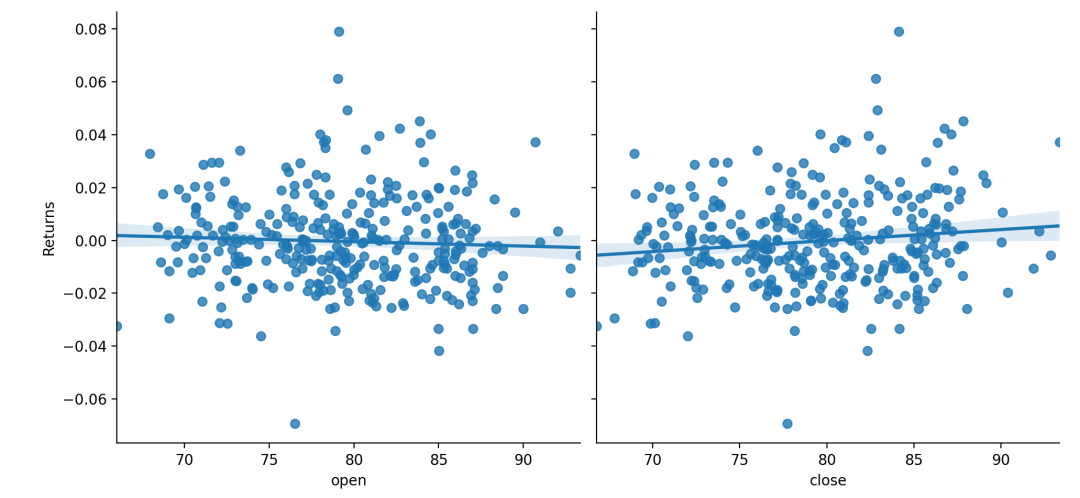-- 数据STUDIO --Python Free

QQ：417803890